﻿ 船舶LNG储槽晃荡热动响应特性分析
 舰船科学技术2020, Vol. 42Issue (8): 82-87    DOI: 10.3404/j.issn.1672-7649.2020.08.015PDF

Thermodynamic response analysis of sloshing ship in marine LNG storage tank
QIAN Xi-hong, CHEN Wei
Merchant Marine College, Shanghai Maritime University, Shanghai 201306, China
Abstract: The LNG ship is affected by the external environment during the voyage, the sloshing of the LNG liquid in the storage tank, and the leakage heat of the maintenance structure of the storage tank, which causes the temperature and pressure in the storage tank to change, and the phase change of LNG leads to the loss of LNG and the safety hazard.. Aiming at the heat and mass transfer process of LNG storage tanks under the influence of sloshing and external heat leakage, a gas-liquid two-phase VOF model was used to establish a mathematical model describing the thermal response of LNG storage tanks, and the leakage and sloshing amplitudes in different externalities were analyzed. And the LNG loss and its heat and mass transfer characteristics in the storage tank and the free surface fluctuation in the LNG storage tank under the height of the LNG liquid level in the storage tank. As the external leakage heat rises, the amplitude and frequency increase, so that the LNG gas zone pressure increase and the LNG loss rate increase, and the increase rate is relatively large as the LNG liquid level in the tank decreases. The analysis results in this paper can provide some theoretical guidance for optimizing the operation of LNG storage tank structure and LNG storage tank.
Key words: LNG storage tank     thermodynamic analysis     sloshing process     low temperature phase transition
0 引　言

1 物理模型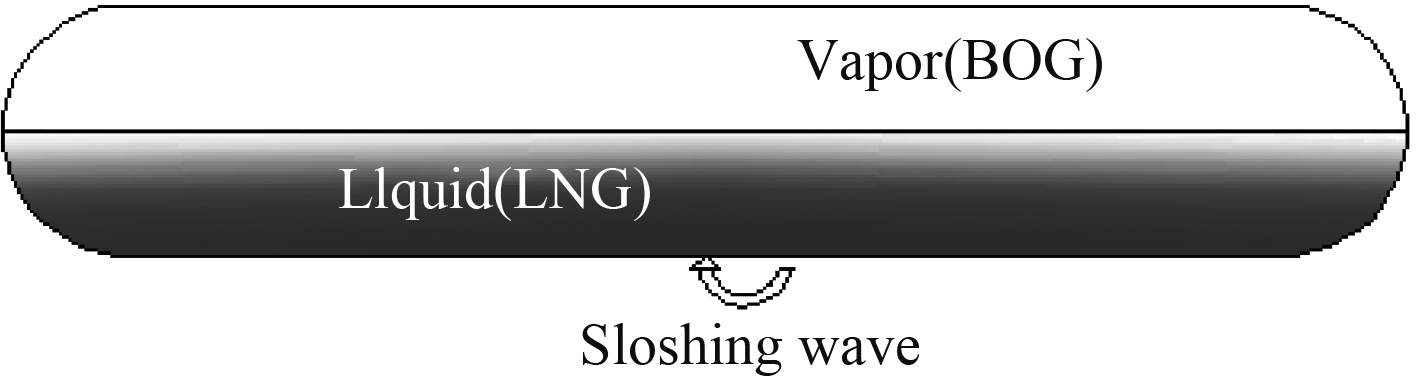图 1 船用LNG储槽二维示意图 Fig. 1 Two-dimensional schematic diagram of a marine LNG storage tank

2 数值模型 2.1 控制方程

LNG储槽内部自由液面受到外部的摇晃会发生移动变化，储槽内存在液相和气相区，利用描述气-液两相流动的VOF模型，分析在晃荡和外界漏热影响下船舶LNG储槽热质传递过程，建立LNG气液相的热动响应及气液界面变化的数学方程如下：

 $\frac{{\partial \rho }}{{\partial t}} + \nabla \left( {\rho \vec v} \right) = {S_{{m}}}\;{\text{，}}$ (1)
 ${\alpha _l} + {\alpha _v} = 1\;{\text{。}}$ (2)

 ${s_m} = \left\{ {\begin{array}{*{20}{c}} {{r_l}{\alpha _l}{\rho _l}\left( {{T_l} - {T_{sat}}} \right) / {T_{sat}},{T_l} \geqslant {T_{sat}}} {\text{，}}\\ {{r_v}{\alpha _v}{\rho _v}\left( {{T_v} - {T_{sat}}} \right)/{T_{sat}},{T_v} < {T_{sat}}} {\text{。}} \end{array}} \right.$ (3)

 ${T_{sat}} = 0.3709{{\rm{e}}^{ - 15}}{p^3} - 3.8013{{\rm{e}}^{ - 10}}{p^2} + 17.956{{\rm{e}}^{ - 5}}p + 96.859{\text{，}}$

 $\begin{split} \frac{\partial }{{\partial t}}\left( {\rho \vec v} \right) + \nabla \left( {\rho \vec v\vec v} \right) =\; & - \nabla p + \nabla \left[ {\mu \left( {\nabla \vec v + \nabla {{\vec v}^{\rm{T}}}} \right)} \right] + \rho g -\\ &\rho ({{\dot \omega }} \times {{r}} + {{\omega }} \times {{\omega }} \times {{r}} + 2{{\omega }} \times {{V}})\;{\text{。}}\end{split}$ (4)

 $\frac{\partial }{{\partial t}}\left( {\rho E} \right) + \nabla \left( {\vec v(\rho E + p)} \right) = \nabla (\lambda \nabla T) + {S_h}\;{\text{。}}$ (5)

 ${S_h} = {S_m}{h_{fg}}\;{\text{。}}$ (6)
2.2 边界条件

 $y = A\sin \left( {\frac{{2{\text{π}}}}{T}t} \right)\;{\text{，}}$ (7)
 $v = y' = A\frac{{2{\text{π}}}}{T}\cos \left( {\frac{{2{\text{π}}}}{T}t} \right)\;{\text{，}}$ (8)
 ${{a}} = y'' = - {{A}}\frac{{4{{\text{π}}^2}}}{{{T^2}}}\sin \left( {\frac{{2{\text{π}}}}{{{T}}}{{t}}} \right)\;{\text{。}}$ (9)

 ${\rm{\lambda }}\frac{{\partial {{T}}}}{{\partial {{x}}}} = {{h}}\left( {{T_a} - {T_w}} \right)\;{\text{。}}$ (10)
3 模型验证和数值计算过程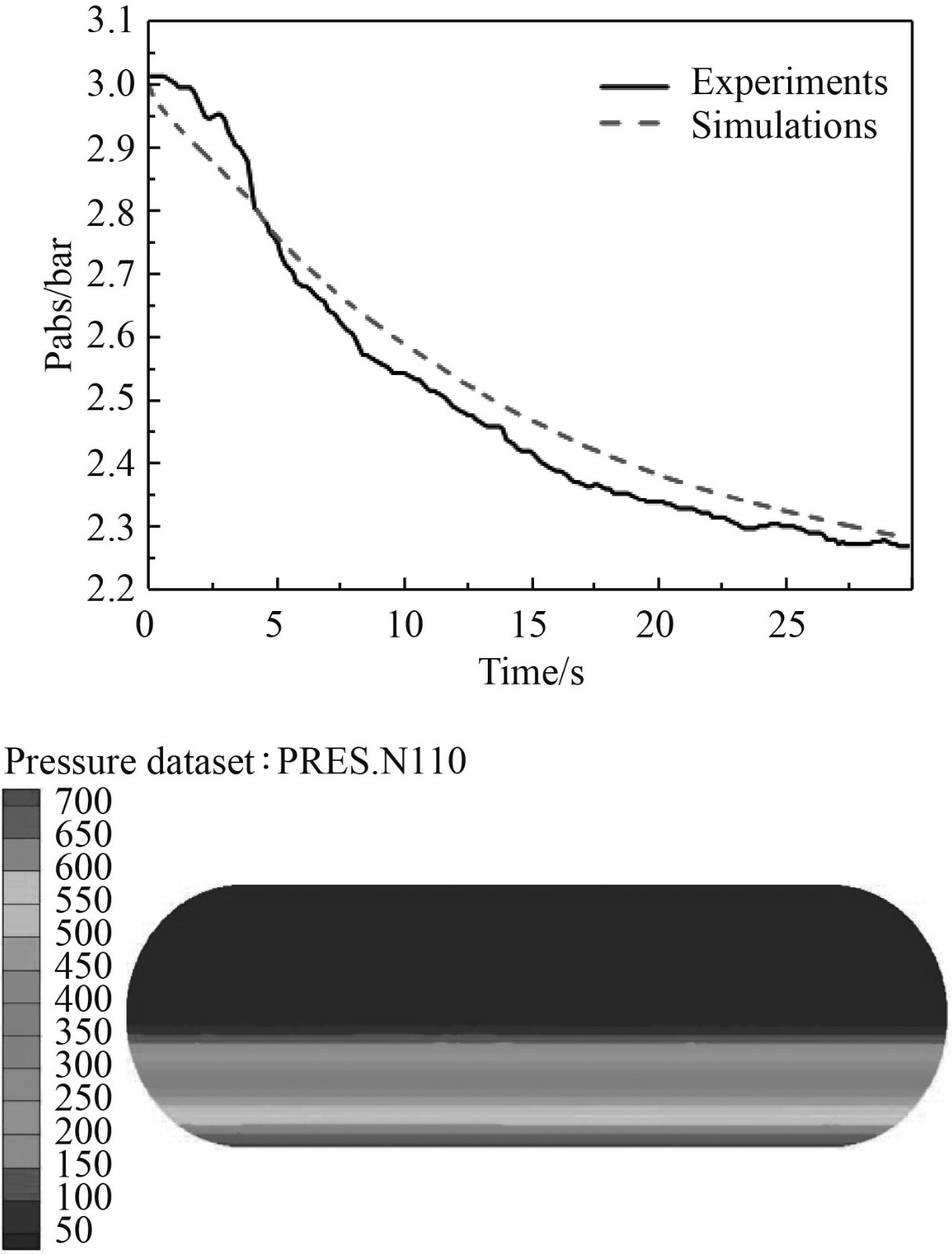图 2 晃荡条储槽内LNG压力计算结果与试验对照 Fig. 2 Calculation result of LNG pressure in sloshing tank and comparison with test
4 结果和讨论 4.1 受晃荡影响储槽LNG液面变化特性

LNG储槽存在气体空间时发生晃荡工况，低温液体与气相区的内界面会诱发连续的波动，本文采取VOF算法计算并捕捉液体表面的形状，为了深入分析波动的变化规律，实验中以气液分界面即整个变化的自由液面为检测面，得出图3中4幅（每幅代表一个晃荡周期）液面抬升的变化曲线图。自由液面的晃荡波动曲线形状在初始液位来回上下变化，左壁面上液位抬升峰值达到0.05 m。从图3（a）可以发现，在–0.2~0 m和0~0.2 m处都出现一个波峰，是由于晃荡动能的传递产生的，从4.2~4.6 s，动态监测点在右侧最高，随着时间的推移，波峰向左侧移动。当波峰到达左侧时，又随着晃荡向右侧推动，自由液面在第1个周期如此往复来回运动。从图3（b）可知，在第2个周期中，自由液面即存在波峰，有出现了波谷。随着晃动能量传递，带动了液体更多的颠簸起伏。图3（c）图3（d）中所示，内界面中的变化又与第1个周期中的类似，可知液体液体吸收了晃动能量之后逐渐趋向稳定了。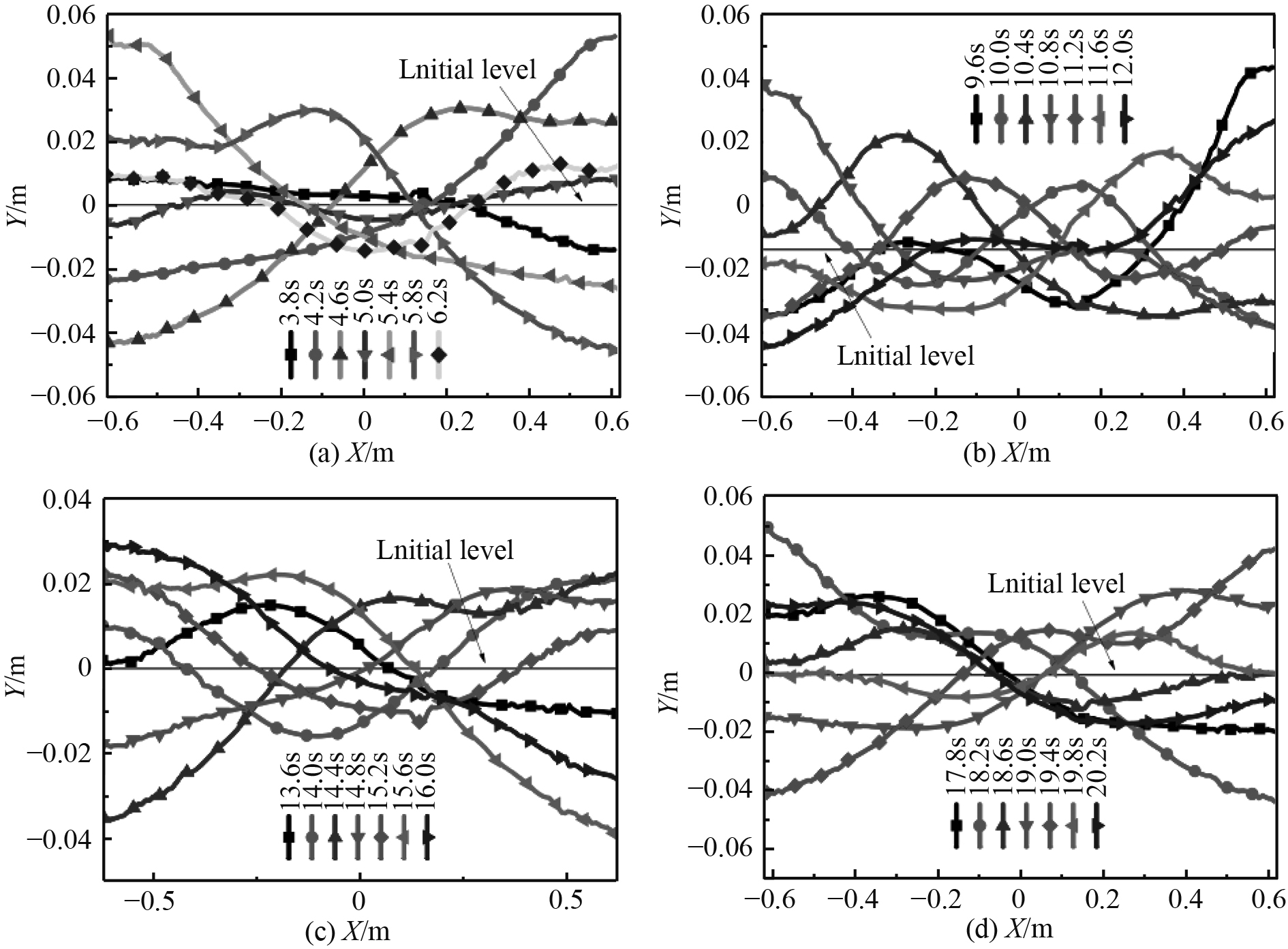图 3 在晃荡条件下储槽内LNG不同时间的液面高度 Fig. 3 Liquid level of LNG in storage tank at different times under sloshing conditions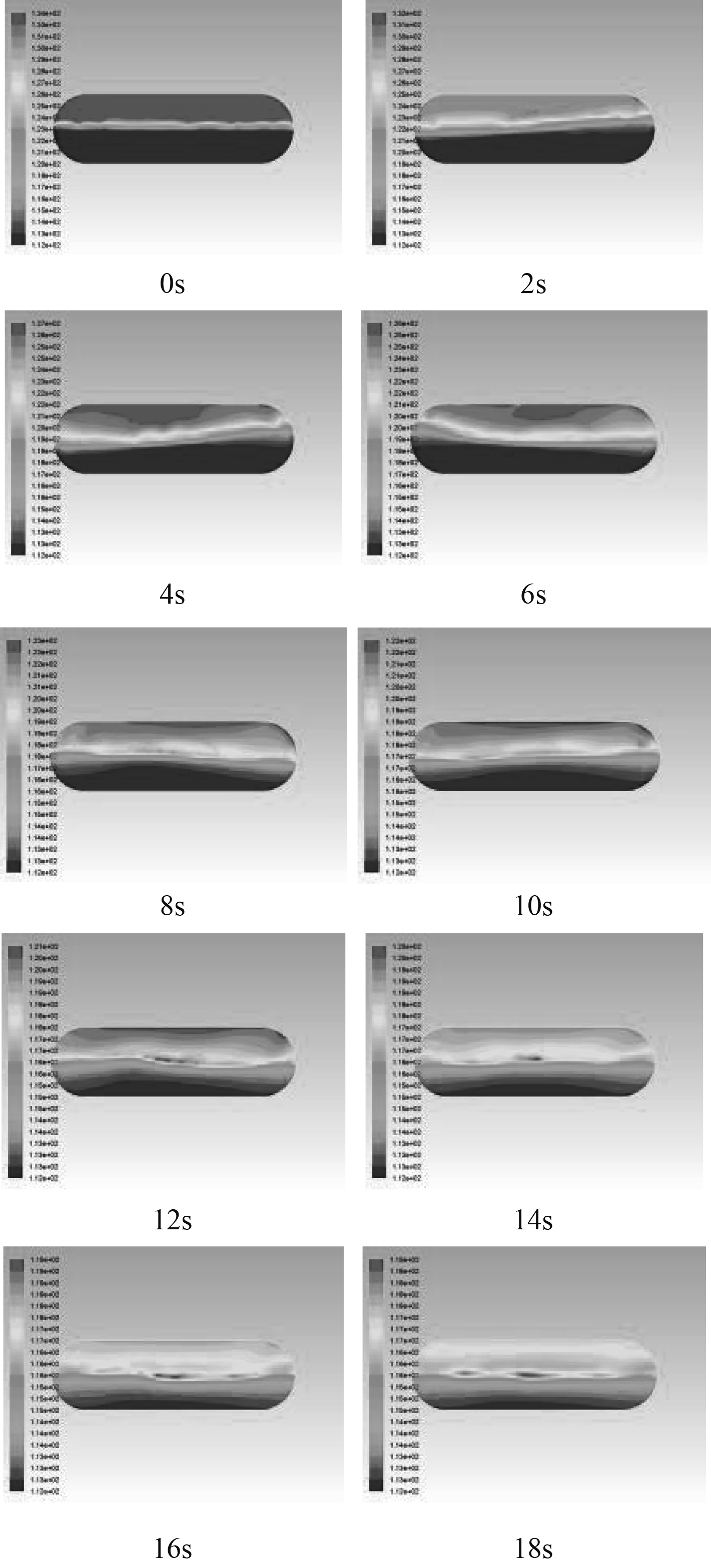图 4 不同时刻下的温度云图 Fig. 4 Temperature contours at different times
4.2 外部热环境影响下晃荡储槽内LNG的热响应特性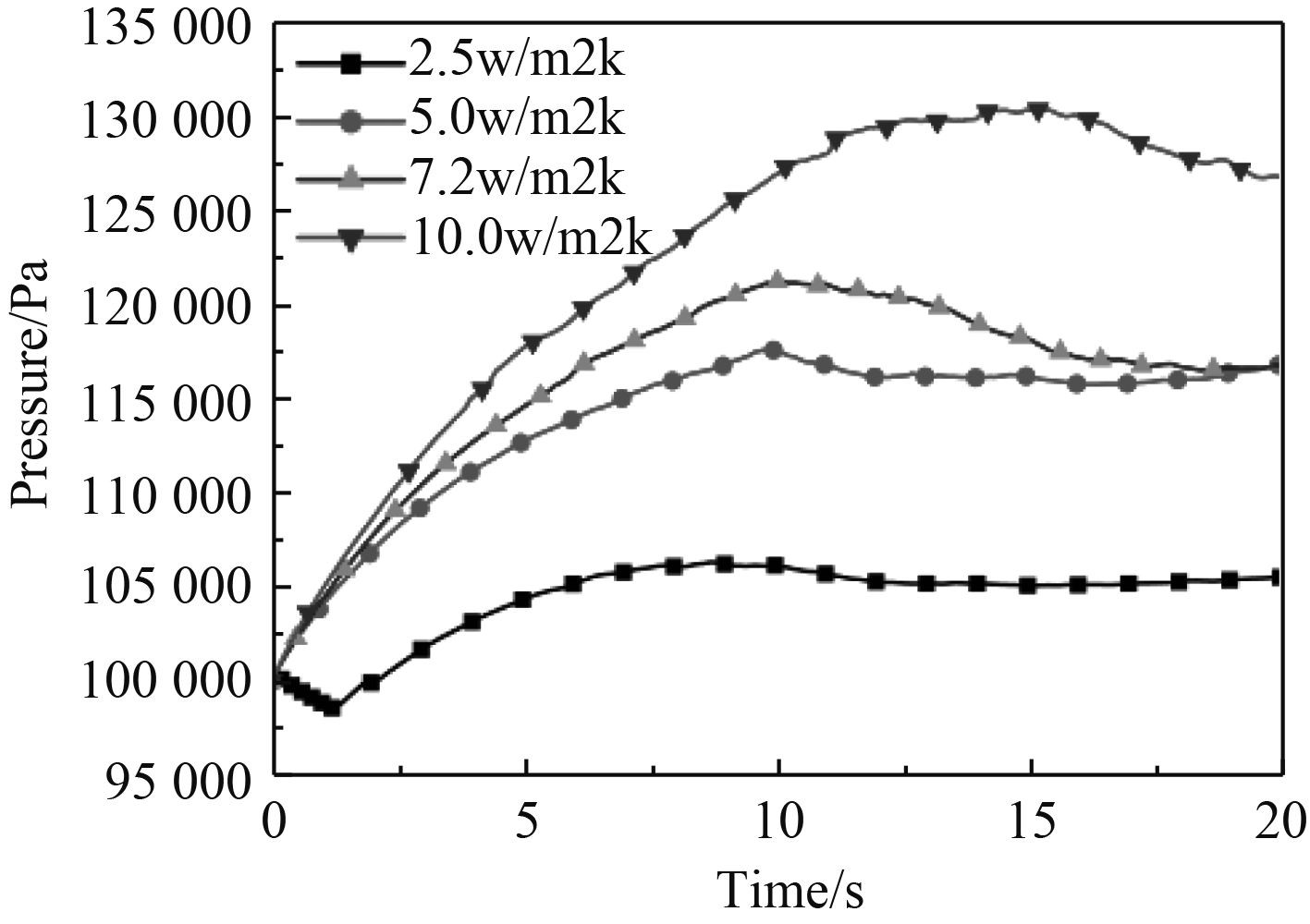图 5 不同对流换热系数下气相区压力随时间的变化 Fig. 5 Variation of pressure in the gas-phase region over time with different convective heat transfer coefficients

4.3 在不同的晃动幅度下储槽内LNG热动响应特性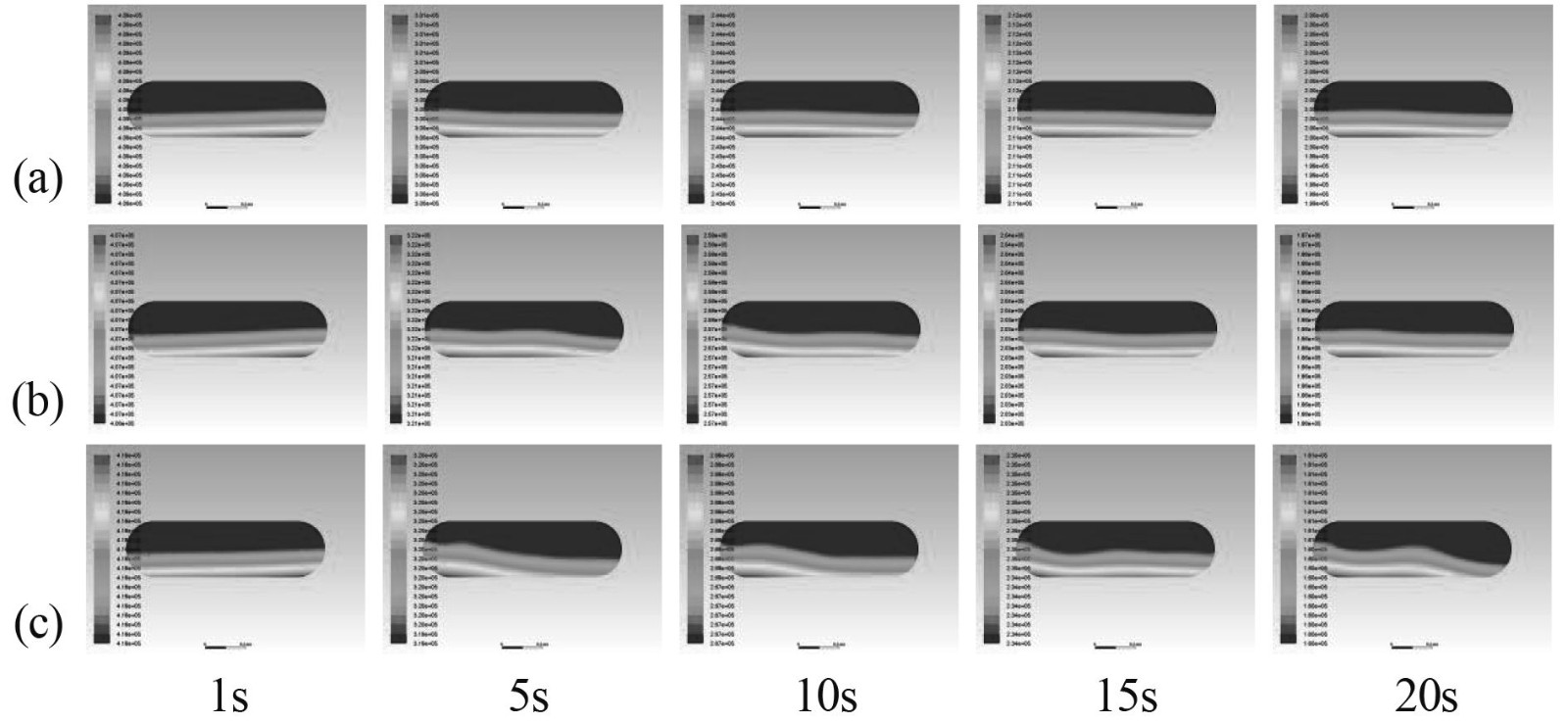图 6 压力分别在A=0.5°（a），A=1°（b），A=2°（c）下不同时刻的压力云图 Fig. 6 Pressure cloud diagrams at different moments of pressure at A = 0.5 °（a），A = 1 °（b） and A = 2°（c）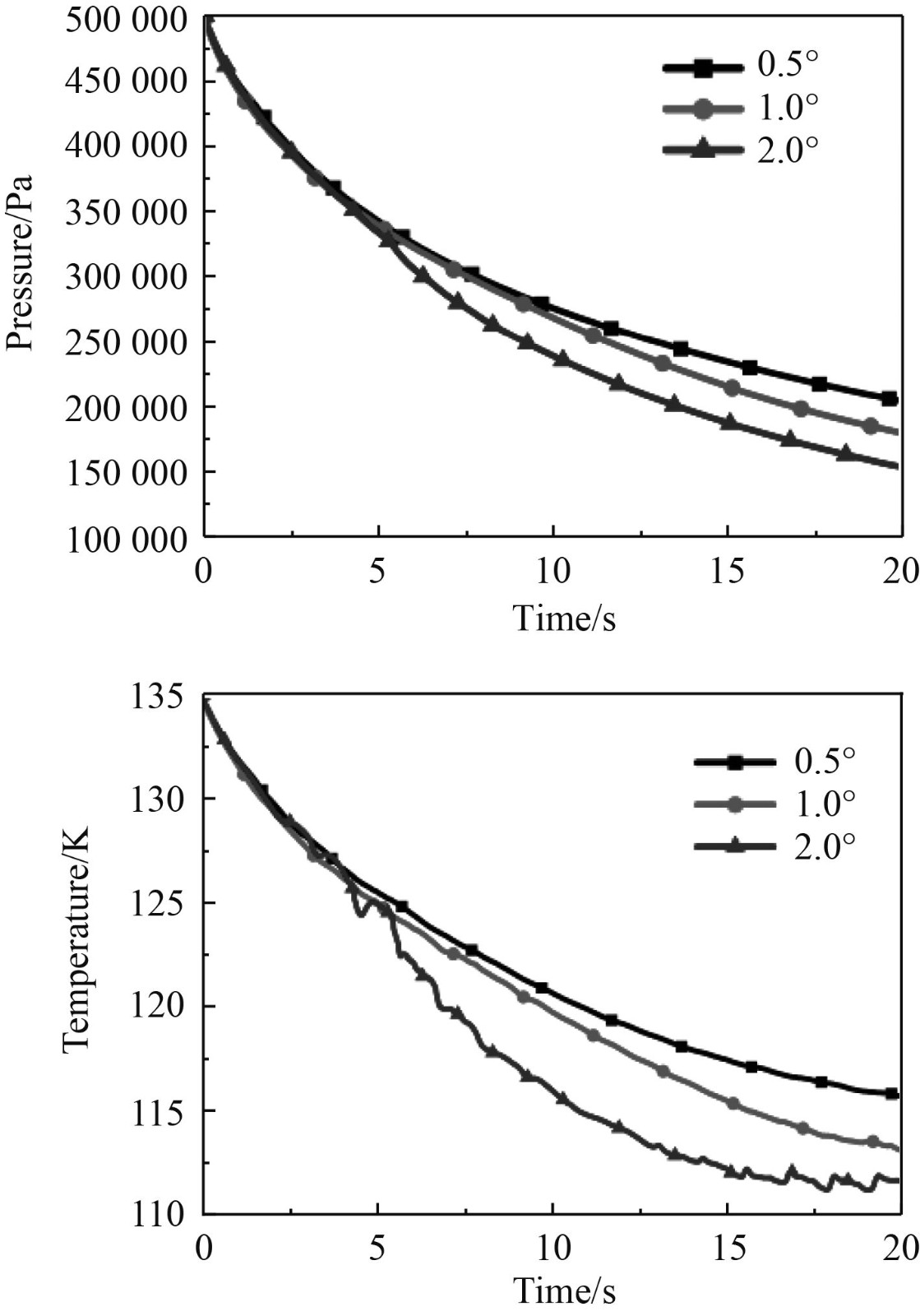图 7 不同振幅下气相区压力和平均温度随时间的变化 Fig. 7 Variations of pressure and average temperature in the gas phase region with time at different amplitudes
4.4 不同LNG液位高度下储槽内压力受晃荡影响特性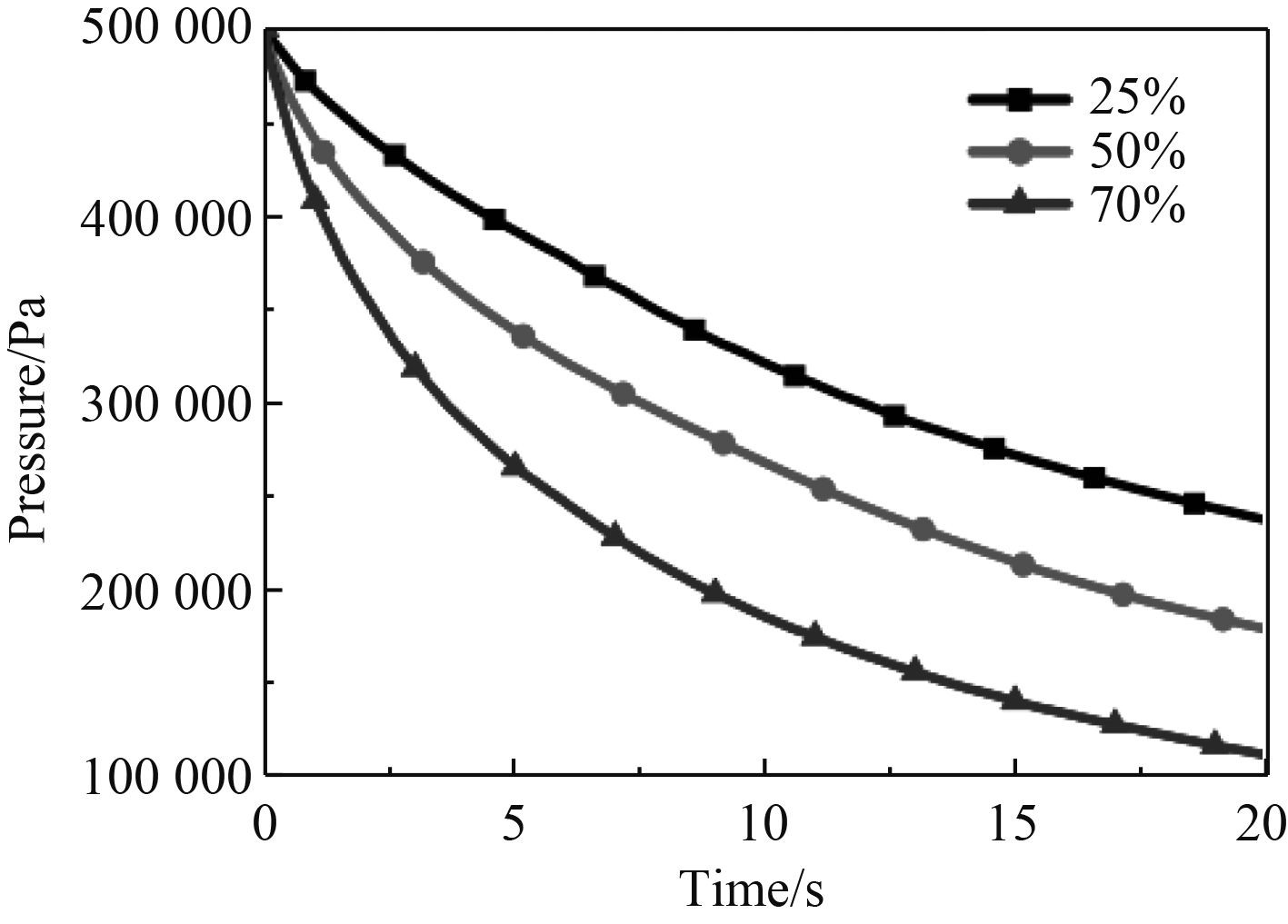图 8 不同充液量下气相区压力变化 Fig. 8 Pressure change in the gas phase region under different filling volumes
5 结　语

  ROH S, SON G, SONG G, et al. Numerical study of transient natural convection in a pressurized LNG storage tank[J]. Applied Thermal Engineering, 2013, 52(1): 209-220. DOI:10.1016/j.applthermaleng.2012.11.021  LEE H B, PARK B J, RHEE S H, et al. Liquefied natural gas flow in the insulation wall of a cargo containment system and its evaporation[J]. Applied Thermal Engineering, 2011, 31(14-15): 2605-2615. DOI:10.1016/j.applthermaleng.2011.04.028  LIU Zhan, FENG, Yuyang, LEI, Gang, et al. (2018). Sloshing hydrodynamic performance in cryogenic liquid oxygen tanks under different amplitudes. Applied Thermal Engineering. 150. 10.1016/j.applthermaleng.2018.12.145.  LEE D H, KIM M H, KWON S H, et al. A parametric sensitivity study on LNG tank sloshing loads by numerical simulations[J]. Ocean Engineering, 2007, 34(1): 3-9. DOI:10.1016/j.oceaneng.2006.03.014  陶文铨. 数值传热学-第2版[M]. 西安: 西安交通大学出版社, 2001.  何晓聪, 何荣. 船用LNG储罐的液体晃荡数值分析[J]. 船海工程, 2016, 45(3). DOI:10.3963/j.issn.1671-7953.2016.03.040  GROTLE E, VILMAR Æsøy. Numerical Simulations of Sloshing and the Thermodynamic Response Due to Mixing[J]. Energies, 2017, 10(9).  李娟. 基于三维参数的船舶稳性设计与优化研究[J]. 舰船科学技术, 2019, 41(02): 14-16. DOI:10.3404/j.issn.1672-7649.2019.02.003